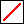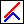# Introduction to MVOModern Portfolio Theory

## Single Period MVO

Mean variance optimization (MVO), also known as Modern Portfolio Theory (MPT), is a quantitative asset allocation technique that allows you to use diversification to balance the risk and return in your portfolio.

Single period MVO was developed in the pioneering work of Markowitz. The goal is to choose a portfolio for the upcoming period. The key concepts introduced by Markowitz were:

• The expected return of the portfolio. This is the "mean" of mean variance optimization.
• The standard deviation of the return on the portfolio, which is treated as a measure of risk. The square of the standard deviation is the "variance" of mean variance optimization.
• The efficient frontier, which is the set of portfolios with expected return greater than any other with the same or lesser risk, and lesser risk than any other with the same or greater expected return.

The efficient frontier is conventionally displayed on a graph with the standard deviation on the horizontal axis and the expected return on the vertical axis. As the assumed level of risk (standard deviation) increases, the obtainable expected return increases. The maximum expected return appears at the right hand end of the frontier, and always consists of a single asset (the one with the highest expected return).

Two advantages of the mean variance formalsim are:

• It simplifies the portfolio selection problem, because the investor need only consider the portfolios on the efficient frontier, rather than the entire universe of possible portfolios.
• It quantifies the notion that diversification reduces risk. Portfolios toward the left end of the efficient frontier can have lower risk than any of the constituent assets.

The efficient frontier graph may be caricatured by the iconof our single period optimizer VisualMvo, in which we see the expected return increasing as more risk is assumed. In practice, the graph is not straight, but rather the slope decreases as the standard deviation becomes larger.

## Multi-period MVO

One problem with the conventional single period analysis is the notion of expected return. More correctly, this should be called the expected single-period return.

If we have historical data, and assume that the future will (in a statistical sense) replicate the past, then the expected return is the simple average (arithmetic mean) of the historical returns in the sample. This is different from the multi-period return (annualized return) of the historical sample, which is given by the so-called geometric mean. The geometric mean is always less than the arithmetic mean. Use of the expected return over-emphasizes the benefit of bearing increasing risk.

The difference between the expected return and the true multi-period return applies both for each individual asset and at the portfolio level. It also applies to the future as well as the past. One might expect that if:

• The investor has selected a portfolio with a given expected return
• The probabaility distribution of returns is stationary (does not change in time)
• In each year the investor rebalances his or her portfolio to the chosen allocation

then the calculated expected return of the portfolio would be the expected annualized return. It is not.

The distinction between the expected return and the long term multi-period return was recognized by Markowitz, but he did not develop a full treatment of multi-period MVO in which the geometric mean could be used as both an input and output of the calculation.

This is achieved in our multi-period optimizer MvoPlus. Here the concepts analogous to those of the single period analysis are:

• The multi-period (geometric mean) return of the portfolio.
• The standard deviation of the return on the portfolio, which is treated as a measure of risk.
• The Geometric Mean Frontier, which is the set of rebalanced portfolios with geometric mean return greater than any other with the same or lesser risk, and lesser risk than any other with the same or greater geometric mean return.

A fundamental difference from the single period case is that the portfolio with the largest geometric mean return is often a diversified portfolio (not just a single asset), and this portfolio can have a geometric mean return that is greater than that of any of the constituent assets.

In a multi-period framework, the mean variance formalism thus has three advantages:

• It simplifies the portfolio selection problem, because the investor need only consider the portfolios on the Geometric Mean Frontier, rather than the entire universe of possible portfolios.
• It quantifies the notion that diversification reduces risk. Portfolios toward the left end of the Geometric Mean Frontier can have lower risk than any of the constituent assets.
• It can actually increase the multi-period return above that of any of the individual assets. This effect is most pronounced when the individual assets have similar multi-period returns.

The multi-period situation may be caricatured by the iconof our multi-period optimizer MvoPlus. Here we see the conventional upward sloping frontier (in red), and the Geometric Mean Frontier (in blue) which can have its maximum for an intermediate value of the standard deviation. This point is sometimes called the "critical point."

The portion of the blue curve to the right of the maximum is not actually part of the Geometric Mean Frontier, but it is displayed by MvoPlus in order to emphasize that increasing the expected return beyond the critical point is actually harmful to the long term return.